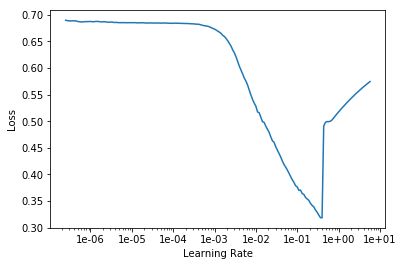# Learning Rate Finder's mathematical theory

My understanding is,
by Learning Rate Finder, we can get an image ‘learning rate’ vs ‘loss’ like this one.And it said,

For a more intuitive explanation, please check out Sylvain Gugger’s post

Then the post How Do You Find A Good Learning Rate (Sylvain Gugger’s post), said,

For a long time, it’s been a game of try and see, but in Cyclical Learning Rates for Training Neural Networks, another approach (I guess it means the mathematical theory of Learning Rate Finder) is presented.

My question is,

• There is no description of Learning Rate Finder in this article (Cyclical Learning Rates for Training Neural Networks), even there is no image ‘learning rate’ vs ‘loss’ in it. Why?
Where does the article describe the problem with the Learning Rate Finder?

• In the process of Learning Rate Finder,
one step (learning rate changes) is synchronized with one mini-batch. Is it right?
If yes: the input image to model is not Invariant, how can we know the change of ‘loss’ is because of learning rate but not the change of input image?
If no: one step (learning rate changes) is synchronized with what?

• In the process of Learning Rate Finder,
Will the weight of the model be updated?
If yes: the ‘loss’ will become smaller and smaller, even ‘learning rate’ doesn’t change.

• The value of ‘loss’ in image ‘learning rate’ vs ‘loss’, is the average of the entire output node, is that right?

I am pretty sure that lr finder has no real mathematical explanation, it is very empirical. They noticed that the lr it choses works very well with 1-cycle policy, at least when tested on kind of simple tasks (and with frozen layers). As for your questions:

• lr steps are indeed synchronized with mini-batches, which doesn’t ensure that variations in loss are due to varations in lr. However:
• with big enough batches, it should not be much of a problem as long as data is not too imbalanced
• You can use as many steps as you want, so that the variation on each batch gets minimal and you mitigate the effect of outliers.
• The goal is to get an overall profile, so we look at a smoothened version of the loss which mitigates the effect of outlier batches.
• The weights get updated (well except those that are frozen), but we want to measure how fast they get changed, which mostly depends on lr. Still, there are other factors, such as the loss profile and indeed the current state of the model.
• No, the value is a smoothened value of loss, taken from an exponential moving average of the loss (with a momentum of 0.98). You can check callback.SmoothenValue to get the formula.

Overall, LRFinder has multiple holes if we try to explain it theoretically but it still gives good empirical results. It doesn’t work all the time though, and is obviously not perfect. Someone from fastai could answer this far better than me however, I am just guessing from the videos I watched and my personal usage of it.

1 Like

I am pretty sure that lr finder has no real mathematical explanation, it is very empirical.

I thought I finally found a mathematical theory method for setting the learning rate, it turns out that it is not…oh…• lr steps are indeed synchronized with mini-batches, which doesn’t ensure that variations in loss are due to varations in lr. However:
• with big enough batches, it should not be much of a problem as long as data is not too imbalanced.
• You can use as many steps as you want, so that the variation on each batch gets minimal and you mitigate the effect of outliers.
• The weights get updated (well except those that are frozen), but we want to measure how fast they get changed, which mostly depends on lr. Still, there are other factors, such as the loss profile and indeed the current state of the model.

I got it.• The goal is to get an overall profile, so we look at a smoothened version of the loss which mitigates the effect of outlier batches.
• No, the value is a smoothened value of loss, taken from an exponential moving average of the loss (with a momentum of 0.98). You can check callback.SmoothenValue to get the formula.

I really don’t know what that, the value is a smoothened value of loss means…smoothened does not equal average?Can you give me an example please?Overall, LRFinder has multiple holes if we try to explain it theoretically but it still gives good empirical results. It doesn’t work all the time though, and is obviously not perfect. Someone from fastai could answer this far better than me however, I am just guessing from the videos I watched and my personal usage of it.

Thanks for your help.You may like to take a look at this post:
Selecting Learn Rates in Fastai Selecting Learn Rates in FASTAI

1 Like

Thanks for your URL.It is an average indeed, an exponentially moving average. Basically, at batch n with momentum \beta, the smoothened loss value s_n associated to loss l_n is s_n = \beta s_{n-1} + (1 - \beta) l_n, with l_0=0. For fastai, \beta = 0.98 which makes the new value of the loss only account for 2% of the actual loss.

1 Like